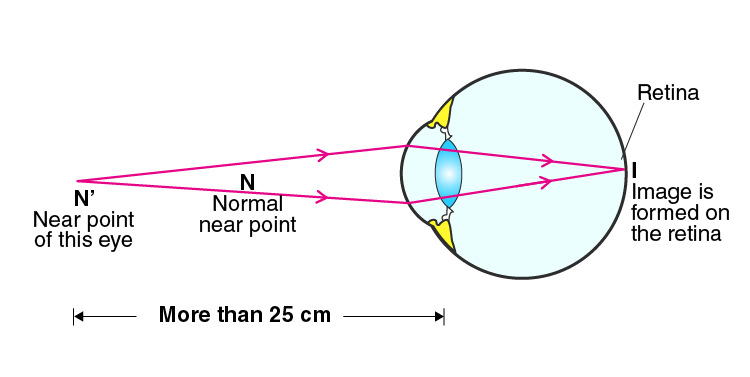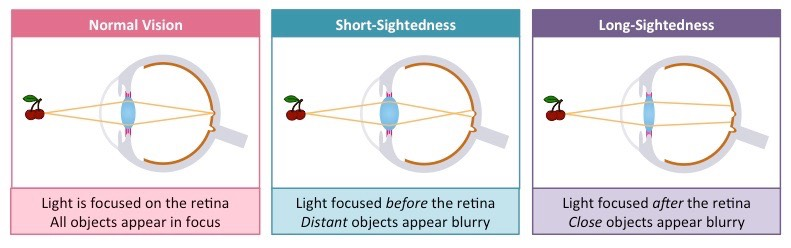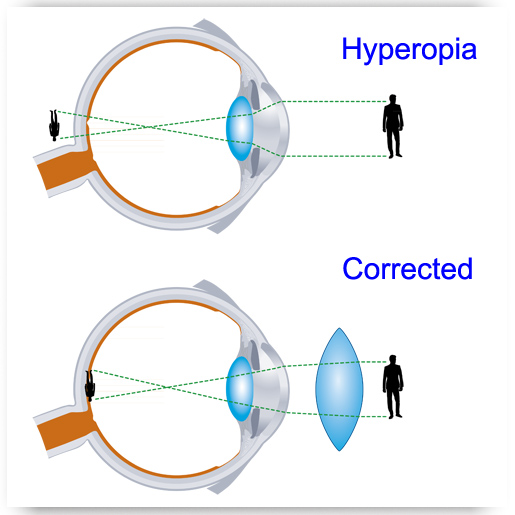# Short Sightedness and Long sightedness

Far and near points

• The eye consists of a lens L and retina R where retina is light sensitive screen.
• Light from the objects enters the eye through the cornea.
• In order to be clearly seen, the image formed by the eye-lens must appear on the retina.
• The ciliary muscles can change the diameter of the lens and hence the focal length of the lens.
• Therefore, the light rays from objects at different distances are focused on the retina.
• This property of eye is called power of accommodation.
• The most distant objects an eye can focus, is called far-point which is at infinity for a normal eye.
• The nearest possible distance at which an object is clearly seen is called least distance of distinct vision (D).
• It is about 25cm for a normal eye and the point at this distance is called near point.Short Sightedness (Myopia)

• In this defect, an eye can see the near objects clearly but is unable to see the distant objects.
• The rays from distant objects are brought to focus at a point in front of the retina.
• This is due to a short focal length of the eye (thick lens) or due to the lengthened eyeball.
• Far point of a myopic eye is not at infinity but lies closer to the eye.

Correction

This defect is corrected using suitable concave lens. The parallel rays from distant objects are made divergent by concave lens. These rays appear to come from the far point P of the defected eye ( or myopic eye). These rays are focused on the retina R, by eye lens. P is image for diverging lens and virtual object for eye lens.

If x is the distance of far point from the eye, then,

For concave lens u =

v= -x (virtual image)

Let f be the focal length of the concave lens, then

1/f = 1/u + 1/v

or, 1/f = 1/∞ + 1/(-x)

f = -x

Hence, the focal length of Concave lens is equal to the distance of the far point from the eye.Image source: atlantaeye

Long Sightedness (Hyper-metropia)

• In this defect, one can see distant objects without strain but the near point is further away from the eye than the normal least distance of distinct vision.
• This defect is due to short eye-ball (thin lens) or long focal length of eye-lens.
• Therefore, rays from the object at normal near point are brought to focus behind the retina.Image source: optometric

Correction

A suitable convex (or converging) lens is used to correct this eye defect. The rays from near point A are converged by extra lens and falls on the eye lens. These rays appear to come from the near point X of the defected eye-lens. Therefore, X is virtual object for eye lens. The final image is formed on the retina R by the eye lens.

Let x is the distance of near point from the eye.

For Convex lens u = D and v = -x

Let f be the focal length of the convex lens.

1/f = 1/u + 1/v

∴ 1/f = 1/D – 1/x

References: